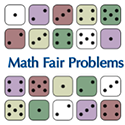# Math Fairs### Math Fair Problems

CLICK HERE for ‘good problems’ for teachers and students to use for Math Fairs and in the general classroom

Dr. Ted Lewis and Dr. Andy Liu of the Mathematics Department, University of Alberta started Math Fairs. Like all good ideas, we borrowed theirs and added a bit of our own personality based on the needs and requests of teachers in our area. We are grateful to them for supporting us.

A Math Fair is a problem-solving fair. Unlike a Science Fair, a Math Fair is all inclusive and noncompetitive. See the guidelines for conducting a Math Fair.

### Math Fair Logistics

Schools are invited to send a team of 4 or 5 teachers to a professional development session. The focus of this meeting is:

• to actively explore a number of ‘good’ problems. These are problems that ask students to look for connections, to develop relationships, to look for patterns, to make conjectures and to develop effective models.
• to provide suggestions for introducing problem-solving and problems to students
• to provide information about hosting a school Math Fair

After this initial introduction, teachers return to their schools with a number of good math problems from the Math Fair booklet, as well as the problems from the Galileo website which they then introduce to their students and school staffs. Dr. Sharon Friesen, Krista Francis-Poscente both of Galileo Educational Network, Dr. Jean Springer, Mathematics Department, University of Calgary, Dr. Eva Nosal, Pacific Institute of Mathematics, and Dr. Indy Lagu, Head of Mathematics Physics and Engineering, Mt. Royal College take turns going to each of the schools participating in Math Fairs for a full day onsite professional development. During the time teachers and students are working on the math problems that they will be presenting at the Math Fair the mathematicians and mathematics educators assist students and teachers to develop extensions to the problems, explore ways to provide hints and ensure that they are comfortable with the Math Fair problems they have designed.

As a culmination to the problem solving work that the students and teachers do, each school hosts a Math Fair at which students set up a display of their math problems, but not the solutions to the problems. Students entice their parents and invited guests to work through their math problems. Mathematicians and mathematics educators are present at this evening event as well to respond to student’s problems and speak with parents about mathematics education.

### Working with Math Fair Problems

#### Day 1: Introduce the Basic Problems to the Students

Prepare and set up 5 stations with a different problem at each station. Have teams of students work through the problems in a team.

NOTE: If this would not work for your students, work through one of the problems first with the whole class and then put them into stations.

#### Day 2: Learning the Problems

Start the day by having students from each group explain the problem they were working on. Have the students explain what was intriguing about each problem, what they had already figured out and what seemed to be confusing. Do NOT let the students jump to solutions.

Have the students move to a second station to try a second problem. (You are building the capacity of the group to figure out some of the math processes they are bringing to bear in each to mathematical reasoning without jumping to answers.)

#### Day 3: Prepare to Teach Each Other

Repeat the start of Day 2.

Have the students choose their favorite of the two problems and prepare the problem for another student in the class. Their job will be to solve the problem they have selected and make it interesting for another student in their class who has not yet had exposure to that problem. They will need to figure out the best way to solve the problem, how to make it interesting and accessible to another person. They will have to decide what manipulatives to make to this problem both interesting and accessible.

#### Day 4: Deconstruct the Learning

– Review each of the problems with the students.
– Compare and analyze the various solutions.
– Discuss the forms of reasoning students brought to the problems.
– Have students discuss various extensions there might be to the problems. How could the problem be made easier? How could it be made more difficult?